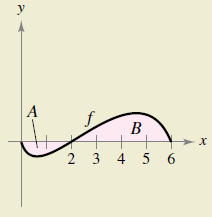Chapter 4.4, Problem 62E

Chapter
Section
Textbook Problem

HOW DO YOU SEE IT? The graph of f is shown in the figure. The shaded region A has an area of 1.5, and ∫ 0 6 f ( x ) d x = 3.5 . Use this information to fill in the blanks.(a) ∫ 0 2 f ( x ) d x =                   (b) ∫ 0 6 f ( x ) d x =                   (c) ∫ 0 6 | f ( x ) | d x =                   (d) ∫ 0 2 − 2 f ( x ) d x =                   (e) ∫ 0 6 [ 2 + f ( x ) ]   d x =                   (f) The average value of f over the interval [0, 6] is                   .(a)

To determine
The blank in the integral represented by, 02f(x)dx=_______

Explanation

Given:

The graph

In the above graph, the shaded region in A, has area 1.5. And the area under complete A+B is 3.5, that is 06f(x)dx=3.5

Explanation:

In the above graph, the shaded area under A depicts where y<0 with area 1.5.

02f(x)dx=1

(b)

To determine
The blank in the integral represented by, 26f(x)dx=_______

(c)

To determine
The blank in the integral represented by, 06|f(x)|dx=_______

(d)

To determine
The blank in the integral represented by, 022f(x)dx=_______

(e)

To determine
The blank in the integral represented by, 06[2+f(x)]dx=_______

(f)

To determine
Theblank in the statement, ‘average value of f over [0,6] is ______.’

Still sussing out bartleby?

Check out a sample textbook solution.

See a sample solution

The Solution to Your Study Problems

Bartleby provides explanations to thousands of textbook problems written by our experts, many with advanced degrees!

Get Started

Find the limit or show that it does not exist. limtt+t22tt2

Single Variable Calculus: Early Transcendentals, Volume I

23/2

Applied Calculus for the Managerial, Life, and Social Sciences: A Brief Approach

In problems 27-30, find. 28.

Mathematical Applications for the Management, Life, and Social Sciences

Fill in each blank: 1kg=g

Elementary Technical Mathematics